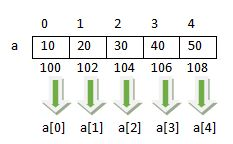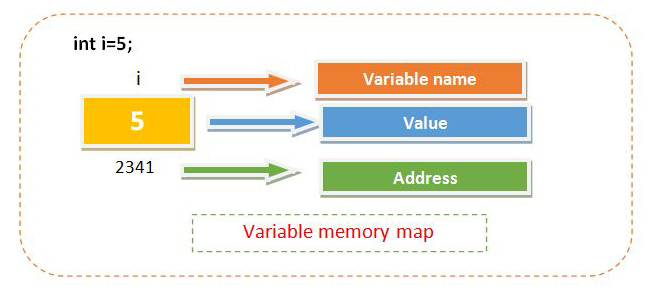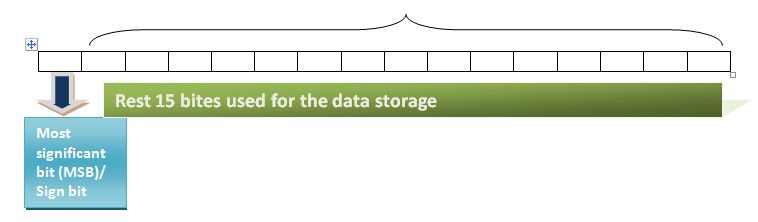## Posts

Showing posts with the label C Programming Tutorial

### Arrays in C LanguageSuppose you want to store the 10 numbers. The one choice is to create a 10 different variable but it is difficult when number of variable are increases. So the next solution that is more feasible is array. An array is the collection of various similar type elements stored in contiguous memory locations. All the array elements share the common name. To uniquely identify each element an index or subscript is associated with the element. so the array is also known as subscript variable. In the given figure arrangement of array elements are shown. The address of the first element is 100. If the given array is of type integer and integer occupies 2 bytes then the next element in the array has address 102,104 etc as given in the figure. How to access array elements using subscript operator it is also shown in the given figure.Array index always start with 0. To access array element subscript operator is used.[] is called subscript operator.a read a of 0 or 0th element of aCreating/Declar…

### RecursionA function is generally call another function to accomplish the task but sometimes a function can call itself to complete the task. This type of function is known as a recursive function and this feature is known as recursion. Let's take a short example of the recursive function.main(){ printf("Hello"); main(); }In the above program snippet main() function calls itself so main() is known as recursive function but the output of the above program snippet is "hello" prints indefinitely. The following program snippet improve the above logic and try to make it finite. main() { int i=1; if(i<=10) { printf("Hello"); i++; main(); } } If you thought that above logic print "Hello" 10 times then you are wrong because when program start i becomes one and of course the condition gets true and "Hello" gets printed then i becomes 2 by increment and then the main() function is again called. As the main()…

### Pointers in C LanguagePointer is called the soul of c. The importance of pointer can be understood by the fact that most of the device driver or embedded system program are created in c. Pointer makes the c most usable and flexible programming. Due to the availability of the pointer, it is possible to write a program such as a device driver. These programs are also known as the device driver. To understand the pointer we have to first understand the memory organization of any variable. The following figure shows the fact. Whenever a variable stored in memory it occupies some memory space and every memory space has a unique number associated with it known as an address. Every byte in member has a different address. If we create an integer variable and suppose it occupy 2 bytes in memory, then two addresses are associated with it but its first address is enough to access it. so the address of next bytes is less useful. To access the address of a variable & (Address of) operator is used. To understand the…

### Functions in C Language

Function is a group of statements which perfoms an specific task.We have used many functions such as printf(),scanf() and many more while developing a program. The purpose of a function can be understood by the example of printf(). Whenever you want to print anything on the output screen you use the printf() function. Actually to send the output on the screen is a difficult task and a program of multiple statements is need to write but fortunately, this is already done by Dennis Ritchie. So you can print anything by using a single line.Types of functionIn c various function are already created such as printf(),scanf(),sqrt(),pow() etc.These are known as predefined function or library function.C also provide the facility to create your own function. The functions created by the programmer are known as user defined function.Every function is followed by a pair of parenthesis(). While working with a function, some input needs to be supplied to the function. The input is given by writing …

### Looping in C Programming

Introduction of loopLooping is a way to repeat a block of statement for the finite number of times. Here finite means that a loop should be terminated automatically or whenever the user wants. We also learn that a program is a collection of instruction. In C there are various control instructions that control the way to execute the program. Sequence control instruction: In which the program statements are executes in the order in which they are written.Decision making control instruction: In which we make a choice by using the if-else construct.Switch/case control instruction: Alternate choice of if-else construct.Loop control instruction: Used to perform the task repeatedly.We have already learned first 3 control instruction, and now we are going to discuss the last one loop control instruction. Let's start the discussion with an example.Suppose you want to print "Hello", then you write print("Hello"); once. But if I tell you, print "Hello" for 100…

### Escape Sequence | Formatted Printing | Interview Questions

Escape sequenceEscape sequence are some non-printable character that shows the effect while printing the output. The usage and example of escape sequence are given below.These are the combination of two characters backslash(\) and one more character but treated as a single character occupy only one byte as other characters.Escape Sequence CharacterMeaningUsage\aBell alertUsed to make a sound.\bbackspaceUsed to move the cursor to the one character back.\nnewlineUsed to change the line\rCarriage ReturnUsed to move the cursor to the beginning of the current line.\"Double QuotesUsed to print the double quotes\'Single QuotesUsed to print the single quotes\fForm feedUsed to change the page\ttabUsed to print the tabProgram to demonstrate the use of escape sequence#include<stdio.h> int main() { printf("Hello\a\a"); printf("Hello\nworld\n"); printf("Hello\tWorld\n"); printf("Hello\"Alex \"\n"); printf("Hello \'Cl…

### Decision Making Statement | Part-2

Check the year is leap year or not.#include<stdio.h> int main() { int year; printf("Enter any year: "); scanf("%d",&year); if(year % 100 == 0) { if (year % 400 == 0) { printf("%d is a leap year",year); } else { printf("%d is not a leap year",year); } }else { if(year % 4 == 0) { printf("%d is a leap year",year); } else { printf("%d is not a leap year",year); } } return 0; } OutputEnter any year: 1996 1996 is leap year Enter any year: 2001 2001 is no a leap year Enter any year: 1800 1800 is not a leap year Program ExplainedYou know the year which is divisible by 4 is called leap year, but what about the century? Each century is divisible by 4. but to check whether the century is a leap year or not we have to check its divisibility by 400. So the logic to check the year is a leap or not:First check the year is a century if the year is century then check its divisibility by 400. if it …

### Decision Making Statements

As in the real world we need to make decision according to the situation. In the same sense we need to make decision while creating program.For decision making C provides various construct as follows:if statementif else statementnested if else statementelse if Laddercondional operatorswitch/case constructBy using the above-given decision-making statement we can implement the sense in a program that program can create decision automatically.if-else statementSyntax-1if(condition) { statement1; statement2; statement3; ........ ........ } else { statement1; statement2; statement3; ........ ........ } Syntax-2if(condition) statement; else statement; Syntax ExplainedThe block which is specified with if is called if-block and the block with else is called else block. If there is a single statement in if block or else block then no need to enclose in {} (curly braces). But in the case of more than one statement and if a…

### Operators in C Language| Part-4

Unary operators Unary operator are those which only require one operand(argument) to operate. + , - , ++, --, typecast and sizeof are some unary operators in C programming. +5, -5 are the example of +,- unary operators. which represents +ve and -ve values.Unary operators have high priority than binary operators, so unary operators are always evalutes first.Increment / Decrement operator++, -- are called increment and decrement operators.Increment and decrement operators are available in two versionsPrefixPostfixDifference b/w prefix and postfixPrefixPostfix int a = 5; ++a; printf("%d",a); // 6 int a = 5; a++; printf("%d",a); // 6 int a = 5,b; b = ++a; printf("%d %d",a,b);// 6 6 int a = 5,b; b = a++; printf("%d %d",a,b);//6 5 Example of increment and decrement operatorsExample-1: int a = 3, b = 4,c; c = ++a * ++b; printf("%d %d %d",a,b,c); //4 5 20 Example-2: int a = 3,b = 4, c; c = a++ * b++; printf("%d %d %d",a,b,c);…

### Operators in C Language | Part-3

Relational OperatorRelational operators are used to perform a logical test or create a condition. List of the logical operator is as given below...Relational opertorMeaning>Greater Than<Less Than>=Greater Than or Equal to<=Less Than or Equal to==Equal to!=Not euqalEvery relational operator returns true or false.In C any non-zero is treated as true and zero is treated as false.In C if any expression evaluated true then system returns 1 otherwise return 0.Relational operator are evaluated from left to right.Example-1 printf("%d",5>2); //print 1 Example-2 printf("%d",5>2>1); //print 0 //first 5>2 evaluated true and returns 1 and then result of this expression is compared with next 1, that is evaluated false. Logical Operator&&(And) ||(Or) !(not) are the 3 logical operators.&&(and), || (or) are used to combine two or more conditions.Each condition can be true or false, so what will be the output of the combination made by logical…

### Operators in C Language | Part-2

Assignment operator= operator is called assignment operator.It is used to move the value or expression result in a variable.Assignment operator are evaluated from right to left order.Example of assignment:a = b = 5, In this example the 5 is assignment in b then b's value is moved to a.a = b + 5, Assignment operator have the lowest precedence so all other operator are evaluated first and then result is moved to the variable.5 = a, This is an error, because in this example we are trying to move the value of variable into constant and constant can't be changed.The same error will be prompted if you are try the expression 6 + b = a.Compound assignment+=, -=, *=, /=, %= are called compound assignment operator. It combine the Arithmetic as well as assignment simultaneously.Compound AssignmentEquivalent Expressiona += 5a = a + 5a -= 5a = a - 5a *= 5a = a * 5a /= 5a = a / 5a %= 5a = a % 5

### Data types in C languageWhat is data type As you have seen in the previous article, a variable is declared as given below...
int a; //variable declaration In the above program code snippet, int is called a data type and a is a variable of that data type.Data type describe the content a variable can store.Data type also explains how data will be stored (the organization) in memory. Data types can be classified into two broad categories... Primitive (Basic) data typeComposite (Secondary) data types / User-defined data types In this article we will only discuss primitive data types, User-defined data types will be discussed later. Primitive data types Data types which are defined by the system/programming language are called primitive data type. These data type defines a single entity, which can't be divided further. List of primitive data types along with their memory occupied and the allowed range are described as below... Integral data typesReal data typesCharacter data typesIntegral data typesData type…